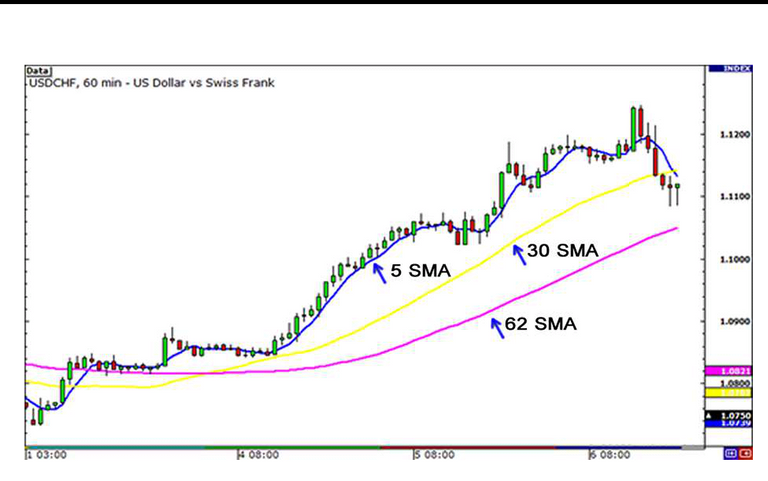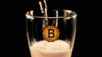in Project HOPE27 days ago (edited)
There is a common saying in trading "the trend is your friend". This implies that you should trade in the direction of the trend. A lot of traders have made a huge amount of profit from following this singular saying. Some traders use tools to make sure they are trading in the direction of the trend while others identify it by just looking at the charts and looking out for higher highs and higher lows or vice versa. Looking for higher highs and higher lows could be very subjective hence the need for an objective way to identify trends. Indicators help with objectivity. The indicators used by traders to objectively identify trends are called trend following indicators. In today's post, I will be writing on the Moving average as an indicator and will base this discussion on simple moving averages.

On this note, I will like to welcome you to another edition of how to grow your trading account.#### What is a moving average?

A moving average is a lagging indicator that shows the average value of a commodity or currency pair over a time period.
In where I come from, when we go to the market we bargain before we buy certain products. The price most buyers bargain for can be likened to the average price of that commodity. when you buy for a higher price, it looks as if you have been cheated.

Some authorities look at moving average as a way to smooth out price action over a period of time.A screendhot showing different moving averages

#### Types of moving average

• Simple moving average
• Exponential moving average
• Weighted moving average

#### Simple moving average

This is a type of moving average that is calculated by simply averaging the price of the currency over a certain period of time. It's as simple as the definition above.

Formula

SMA=(P1 + P2 + Pn)/n

Where

P= Price
n =number of days or periods

Example:
If the price of EUR/USD was 1.5000, 1.5199, 1.5200, 1.5000, and 1.5120 over the last 5 days. Calculate the simple moving average.

Solution
Step 1

1.500 + 1.5199 + 1.5200 + 1.5 + 1.5120 = 7.5519

Step 2
Divide the total by the total number of days

7.5519/5=1.5104

The simple moving average is 1.5104

#### Significance of simple moving average.

Knowing how to calculate without knowing the importance of SMA is disastrous. Simple moving averages are one of the easiest indicators to use and every trader should be able to use and interpret their signals.
When the price is trading above the simple moving average it simply means that there is an uptrend and if the price is trading below the simple moving average it means the trend is down. Easy right?

Is simple moving average a perfect indicator?
The answer is a straight forward NO. The problem with SMA is the fact that price changes two times. First, if a price is dropped and when a price is added. If there was a spike in price due to one news or the other the price reacts so fast for a short time period and changes and so the average might not be an actual reflection of the trend. Also if a price is also removed there is a huge change in the price if the price was significantly higher or lower than the current average. We trade in the present and we want something that reflects more of the present and not much of the past. This is where the SMA is flawed.

When an old piece of data gets dropped off, a simple moving average jumps. This problem is worse with short MAs but not so bad with long MAs. If you use a 10-day MA, those drop-offs can really shake it because each day constitutes 10% of the total value. On the other hand, if you use a 200-day MA, where each day is responsible for only 0.5%, dropping off a day isn’t going to influence it a lot.

This is an excerpt from the new trading for a living by Alexander Elder. This excerpt analyses the downfall of using SMA and it shows that SMAs can be misleading when the time period is short. Hence, the need for an average that is sensitive to the current market situation.

In my next post, I will be writing on the Exponential moving average. A moving average that solves one of the problems of a simple moving average.

#### References

1. Babypips
2. Investopedia
3. wikipedia
4. pixabay
5. The new trading for a living by Dr. Alexander Elder
Sort:

!BEERView or trade `BEER`.

Hey @bhoa, here is a little bit of `BEER` from @gamsam for you. Enjoy it!

Learn how to earn FREE BEER each day by staking your `BEER`.

@tipu curate

Congratulations @bhoa! You have completed the following achievement on the Hive blockchain and have been rewarded with new badge(s) :You distributed more than 6000 upvotes. Your next target is to reach 7000 upvotes.

If you no longer want to receive notifications, reply to this comment with the word `STOP`Hive Power Up Day - Introducing the Power Up Helper!